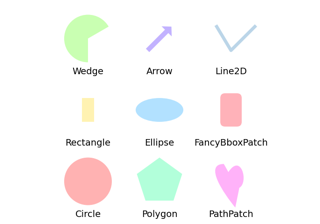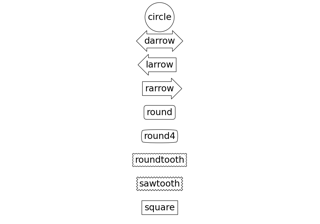# matplotlib.patches.BoxStyle¶

class matplotlib.patches.BoxStyle[source]

Bases: matplotlib.patches._Style

BoxStyle is a container class which defines several boxstyle classes, which are used for FancyBboxPatch.

A style object can be created as:

BoxStyle.Round(pad=0.2)


or:

BoxStyle("Round", pad=0.2)


or:

BoxStyle("Round, pad=0.2")


The following boxstyle classes are defined.

Class Name Attrs
Circle circle pad=0.3
DArrow darrow pad=0.3
LArrow larrow pad=0.3
RArrow rarrow pad=0.3
Round round pad=0.3, rounding_size=None
Round4 round4 pad=0.3, rounding_size=None
Roundtooth roundtooth pad=0.3, tooth_size=None
Sawtooth sawtooth pad=0.3, tooth_size=None
Square square pad=0.3

An instance of any boxstyle class is an callable object, whose call signature is:

__call__(self, x0, y0, width, height, mutation_size, aspect_ratio=1.)


and returns a Path instance. x0, y0, width and height specify the location and size of the box to be drawn. mutation_scale determines the overall size of the mutation (by which I mean the transformation of the rectangle to the fancy box). mutation_aspect determines the aspect-ratio of the mutation.

Return the instance of the subclass with the given style name.

class Circle(pad=0.3)[source]

Bases: matplotlib.patches._Base

A circular box.

__init__(self, pad=0.3)[source]

Initialize self. See help(type(self)) for accurate signature.

__module__ = 'matplotlib.patches'
transmute(self, x0, y0, width, height, mutation_size)[source]

Return the Path outlining the given rectangle.

class DArrow(pad=0.3)[source]

Bases: matplotlib.patches._Base

A box in the shape of a two-way arrow.

__init__(self, pad=0.3)[source]

Initialize self. See help(type(self)) for accurate signature.

__module__ = 'matplotlib.patches'
transmute(self, x0, y0, width, height, mutation_size)[source]

Return the Path outlining the given rectangle.

class LArrow(pad=0.3)[source]

Bases: matplotlib.patches._Base

A box in the shape of a left-pointing arrow.

__init__(self, pad=0.3)[source]

Initialize self. See help(type(self)) for accurate signature.

__module__ = 'matplotlib.patches'
transmute(self, x0, y0, width, height, mutation_size)[source]

Return the Path outlining the given rectangle.

class RArrow(pad=0.3)[source]

Bases: matplotlib.patches.LArrow

A box in the shape of a right-pointing arrow.

__init__(self, pad=0.3)[source]

Initialize self. See help(type(self)) for accurate signature.

__module__ = 'matplotlib.patches'
transmute(self, x0, y0, width, height, mutation_size)[source]

Return the Path outlining the given rectangle.

class Round(pad=0.3, rounding_size=None)[source]

Bases: matplotlib.patches._Base

A box with round corners.

__init__(self, pad=0.3, rounding_size=None)[source]

Initialize self. See help(type(self)) for accurate signature.

__module__ = 'matplotlib.patches'
transmute(self, x0, y0, width, height, mutation_size)[source]

Return the Path outlining the given rectangle.

class Round4(pad=0.3, rounding_size=None)[source]

Bases: matplotlib.patches._Base

A box with rounded edges.

__init__(self, pad=0.3, rounding_size=None)[source]

Initialize self. See help(type(self)) for accurate signature.

__module__ = 'matplotlib.patches'
transmute(self, x0, y0, width, height, mutation_size)[source]

Return the Path outlining the given rectangle.

class Roundtooth(pad=0.3, tooth_size=None)[source]

Bases: matplotlib.patches.Sawtooth

A box with a rounded sawtooth outline.

Parameters: padfloat, default: 0.3The amount of padding around the original box. tooth_sizefloat, default: pad/2Size of the sawtooth.
__init__(self, pad=0.3, tooth_size=None)[source]

Initialize self. See help(type(self)) for accurate signature.

__module__ = 'matplotlib.patches'
transmute(self, x0, y0, width, height, mutation_size)[source]

Return the Path outlining the given rectangle.

class Sawtooth(pad=0.3, tooth_size=None)[source]

Bases: matplotlib.patches._Base

A box with a sawtooth outline.

Parameters: padfloat, default: 0.3The amount of padding around the original box. tooth_sizefloat, default: pad/2Size of the sawtooth.
__init__(self, pad=0.3, tooth_size=None)[source]

Initialize self. See help(type(self)) for accurate signature.

__module__ = 'matplotlib.patches'
transmute(self, x0, y0, width, height, mutation_size)[source]

Return the Path outlining the given rectangle.

class Square(pad=0.3)[source]

Bases: matplotlib.patches._Base

A square box.

__init__(self, pad=0.3)[source]

Initialize self. See help(type(self)) for accurate signature.

__module__ = 'matplotlib.patches'
transmute(self, x0, y0, width, height, mutation_size)[source]

Return the Path outlining the given rectangle.

__module__ = 'matplotlib.patches'

## Examples using matplotlib.patches.BoxStyle¶